﻿ 文章 - ApeNixX

Java实现六种经典排序算法

## 二、七种经典排序

### 1. 冒泡排序

`时间复杂度：最好时间：O（n） 最坏：O（n^2） 平均：O（n^2）`

`辅助空间：O（1）`

`稳定性：稳定`

``````public static int [] bubbleSort(int a[]){
int  temp;
for(int i=0;i<a.length-1;i++){
for(int j=0;j<a.length-i-1;j++){
if (a[j] > a[j+1]) {  //前一个比后一个大，就进行交换
temp = a[j];
a[j] = a[j+1];
a[j+1] = temp;
}
}
}
return  a;
}
``````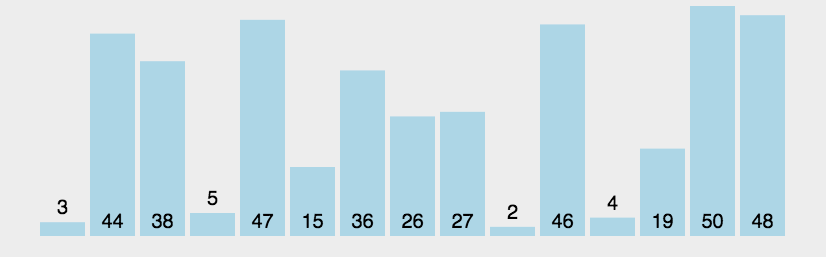### 2.直接选择排序

`时间复杂度：最好 最坏 平均时间均为O（n^2）` `辅助空间：O（1）` `稳定性：不稳定`

`````` private static int[] chooseSort(int[] a) {
for (int i=0;i<a.length-1;i++){
int  minIndex = i;
for(int j=i+1;j<a.length;j++){
if(a[j] < a[minIndex]){//找到最小的数
minIndex  = j;//将最小数的索引保存
}
}

int temp = a[i];
a[i] = a[minIndex];
a[minIndex] = temp;

/*-------------------此段代码只是为了研究插入排序运行流程而添加-----------------------*/
for (int k = 0; k < a.length; k++) {
System.out.print(a[k]+"   ");
}
System.out.println();
/*-------------------此段代码只是为了研究插入排序运行流程而添加-----------------------*/

}
return  a;
}
``````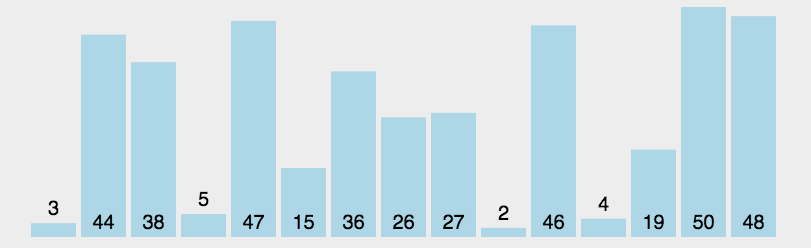### 3.直接插入排序

算法思想：

• 初始时假设第一个记录自成一个有序序列，其余记录均为无序序列
•  接着从第二个记录开始，按照记录的大小依次将当前处理的记录插入到其之前的有序序列中
• 直到最后一个记录插入到有序序列中为止

`时间复杂度：最好时间：O（n）；最坏：O（n^2） 平均：O（n^2）`   `辅助空间：O（1）`  `稳定性：稳定`

``````  public static int [] insertSort(int a[]){
int insertNode;
int leftindex;
for(int i=1;i<a.length;i++){
insertNode = a[i];//用作比较的数据
leftindex  = i-1;
while(leftindex >=0 && a[leftindex]>insertNode){//当比到最左边或者遇到比insertNode小的数据时，结束循环
a[leftindex +1] = a[leftindex];//将左边的值赋给右边
leftindex --;
}
a[leftindex+1] = insertNode;//把要比较的数插入到合适的位置
/*-------------------此段代码只是为了研究插入排序运行流程而添加-----------------------*/
for (int k = 0; k < a.length; k++) {
System.out.print(a[k]+"   ");
}
System.out.println();
/*-------------------此段代码只是为了研究插入排序运行流程而添加-----------------------*/
}
return  a;
}
``````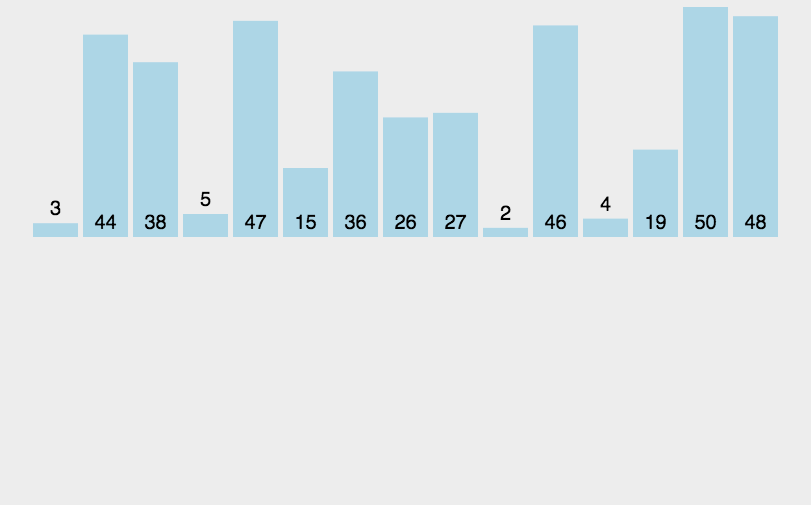### 4.快速排序

思想：  `分治法` ① 对于一组给定的记录，通过一趟排序后，将原序列分为两部分，其中前一部分的所有记录均比后一个序列的记录小； ② 然后再依次对前后两部分的记录进行快速排序

`时间复杂度：最坏时间：O（n^2）； 最好和平均：O（n*log n） 辅助空间：O（log n）`  `稳定性：不稳定`

**  快排是所有平均时间复杂度为O（n*log n）算法中，平均性能最好的！！**

``````public class QuickSort {

public static void main(String[] args) {
int[] a = {1, 2, 4, 5, 7, 4, 5 ,3 ,9 ,0};
System.out.println(Arrays.toString(a));
quickSort(a);
System.out.println(Arrays.toString(a));
}

public static void quickSort(int[] a) {
if(a.length>0) {
quickSort(a, 0 , a.length-1);
}
}

private static void quickSort(int[] a, int low, int high) {
//1,找到递归算法的出口
if( low > high) {
return;
}
//2, 存
int i = low;
int j = high;
//3,key
int key = a[ low ];
//4，完成一趟排序
while( i< j) {
//4.1 ，从右往左找到第一个小于key的数
while(i<j && a[j] > key){
j--;
}
// 4.2 从左往右找到第一个大于key的数
while( i<j && a[i] <= key) {
i++;
}
//4.3 交换
if(i<j) {
int p = a[i];
a[i] = a[j];
a[j] = p;
}
}
// 4.4，调整key的位置
int p = a[i];
a[i] = a[low];
a[low] = p;
//5, 对key左边的数快排
quickSort(a, low, i-1 );
//6, 对key右边的数快排
quickSort(a, i+1, high);
}

}
``````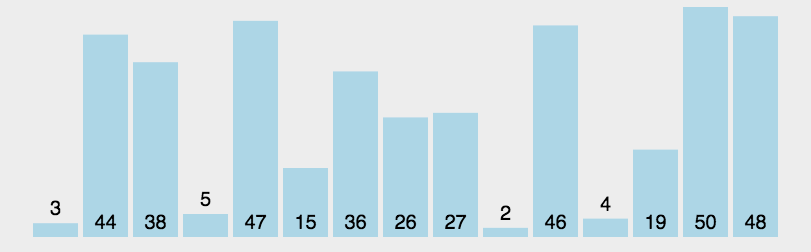### 5.希尔排序

希尔排序也叫“缩小增量排序”,是插入排序的改进版   思想： ①.首先选择一个步长序列，t1=n/2, t2=t1/2, ... ,tk=1；如n=10,则步长序列{5,2,1} ②.然后按步长序列个数k，对待排序序列进行k趟排序； ③.每趟排序，根据对应步长ti,将待排序列分成ti个子序列，分别对各个子序列进行直接插入排序。

`时间复杂度：最坏 平均：O（n*log n）也有地方写O（n^1.25）`   `辅助空间：O（1）`  `稳定性：不稳定`

``````public class ShellSort {

public static void shellsort2(int a[], int n)
{
int j, gap;

for (gap = n / 2; gap > 0; gap /= 2)
for (j = gap; j < n; j++)//从数组第gap个元素开始
if (a[j] < a[j - gap])//每个元素与自己组内的数据进行直接插入排序
{
int temp = a[j];
int k = j - gap;
while (k >= 0 && a[k] > temp)
{
a[k + gap] = a[k];
k -= gap;
}
a[k + gap] = temp;
}
}

public static void main(String[] args) {
int[] array = {99,-1,5,4,3,7,6,8,10,1};
System.out.print("原数组：");
for(int ai : array) {
System.out.print(ai+" ");
}
System.out.println();

shellsort2(array,array.length);

System.out.print("希尔排序后：");
for(int ai : array) {
System.out.print(ai+" ");
}

}

}

``````### 6.归并排序

思想：  利用递归与分治技术将数据序列划分为越来越小的半子表，再对半子表排序，最后用递归将排好序的半子表合并为越来越大的有序序列。 ① “归”--表示递归，即递归将数组折半地分为单个数组 ② “并”--表示合并，即将分开的数据按照从小到大或从大到小的顺序再放到一个数组中  ③对于给定一组记录，首先将每两个相邻的长度为1的子序列进行归并，得到【n/2】(向上取整)个长度为2或者1的有序子序列，再将其两两归并；反复执行此过程直到得到一个有序序列

`时间复杂度：最好、 最坏、 平均时间：O（n*log n）`  `辅助空间：O（n）`                         `稳定性：稳定`

``````public class MergeSort {
/*
* 1.将两个有序数组进行合并，a[first,mid] 和 a[mid+1,last]
*/
public static void mergeArray(int[] a, int first, int mid, int last, int[] temp){
int i = first, j = mid+1; 	//设置两个数组的起始下标
int m = mid, n = last; 		//设置两个数组结束下标,俩数组变为a[i,m],a[j,n]
int k = 0; 					//辅助数组temp[]的下标

while(i<=m && j<=n) { 		//两个数组均未到达末尾时,每次将较小的值赋给temp[k]
if(a[i] <= a[j]) {
temp[k++] = a[i++];
}else {
temp[k++] = a[j++];
}
}

while(i <= m) {	//第一个数组还有数据，第二个数组完事了，直接将第一个剩下的复制到temp中
temp[k++] = a[i++];
}

while(j <= n) {	//第二个数组还有数据，第1个数组完事了，直接将第2个剩下的复制到temp中
temp[k++] = a[j++];
}

for(i=0 ; i<k ; i++) {
a[first+i] = temp[i];	//将排好序的部分复制回去原数组，即更新原数组a[first,last]
}
}

/*
* 2.二路归并排序，递归实现
*/
public static void mergeSort(int[] a, int first, int last, int[] temp) {
if(first < last) {
int mid = (first + last)/2;

mergeSort(a, first, mid, temp); //将原数组左半部分排序，递归

mergeSort(a, mid+1, last, temp); //将原数组右半部分排序，递归

mergeArray(a, first, mid, last, temp); //合并两个有序的数组
}
}

public static void main( String[] args ) {
int[] array = {99,5,4,3,7,6,8,10,-1,1};
int[] temp = {0,0,0,0,0,0,0,0,0,0};
System.out.print("原数组：");
for(int ai : array) {
System.out.print(ai+" ");
}
System.out.println();

mergeSort(array,0,array.length-1,temp);

System.out.print("归并排序后：");
for(int ai : array) {
System.out.print(ai+" ");
}
}
}

``````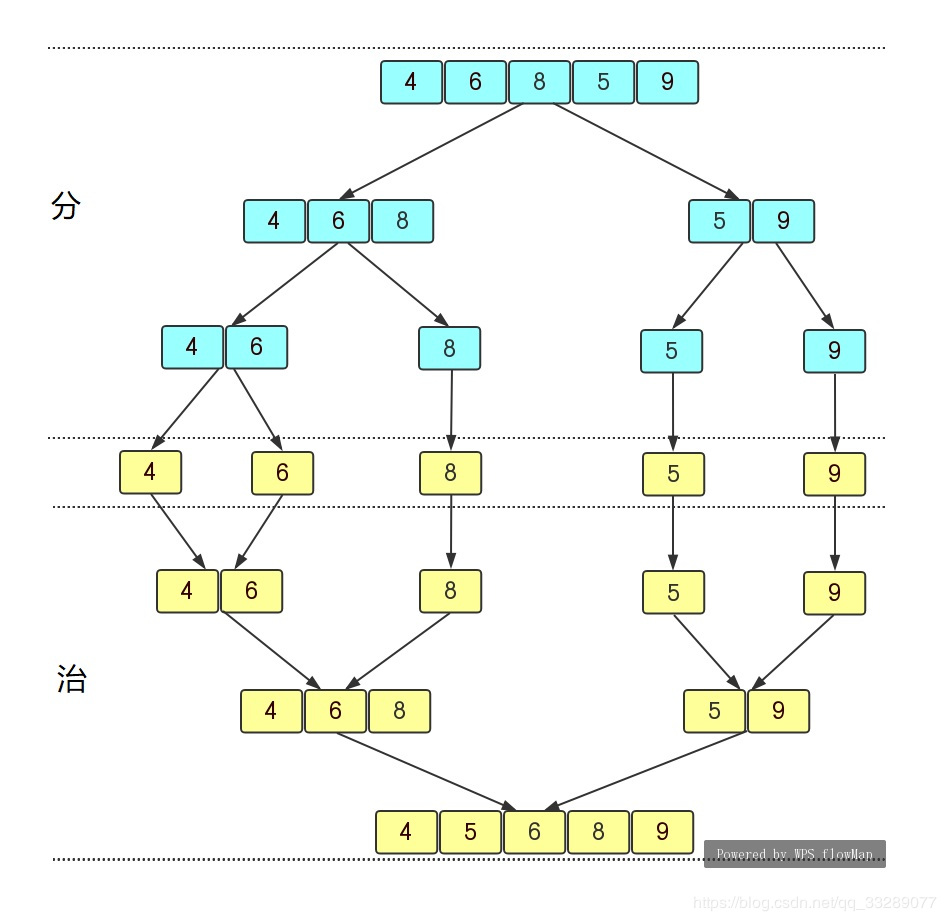## 三、总结

1. 插入排序包括：直接插入排序、希尔排序

2. 选择排序包括：直接选择排序、堆排序

3. 交换排序包括：冒泡排序、快速排序

4. 归并排序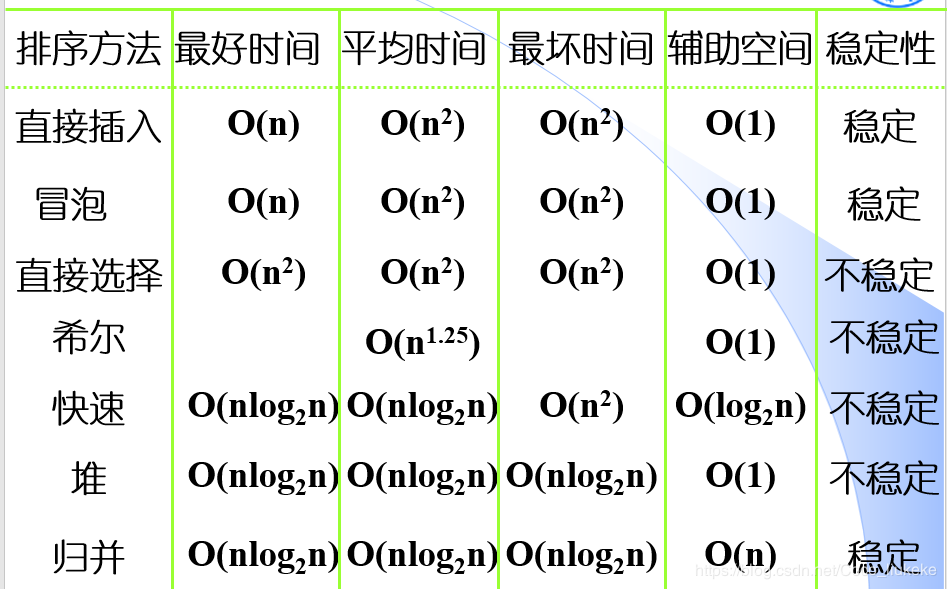面试    排序    算法

•不落阁
我为大家做了模拟留言与回复！试试吧！Absolutely这是用户回复内容

2017-03-18 18:26回复Absolutely 回复 不落阁这是第二个用户回复内容

2017-03-18 18:26回复